Nuclear Science
Volume 2, Issue 1, March 2017, Pages: 11-15

Review Article

Estimation of Semi-Empirical Mass Formula Coefficients

1Faculty of Physics, Shahid Bahonar University of Kerman, Kerman, Iran

2Department of Physics, Payame Noor University, Tehran, Iran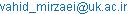(M. M. A. Vahid)

*Corresponding author

Mirzaei Mahmoud Abadi Vahid, Mirhabibi Mohsen, Askari Mohammad Bagher. Estimation of Semi-Empirical Mass Formula Coefficients. Nuclear Science. Vol. 2, No. 1, 2017, pp. 11-15. doi: 10.11648/j.ns.20170201.13

Received: December 26, 2016; Accepted: January 12, 2017; Published: February 4, 2017

Abstract: Using linear least squares method and by data of atomic mass, the present study calculates the coefficients of volume, surface, Coulomb, and asymmetry terms in semi-empirical formula. Our findings show that the mass of neutron and hydrogen can be estimated via developing this example. The results of the present calculations are also compared with those of similar previous studies.

Keywords: Liquid Drop Model, Semi Empirical Mass Formula, Least Square Method, Data Fitting, Experimental Data

Contents

1. Introduction

In the area of nuclear physics model based on experimental data such as is common to many fields. These models have been confirmed by experimental data and must able to predict some other data. One of the basic and important models in nuclear physics is liquid drop model (LDM). The essential assumptions of this model are

a.  The nucleus consists of incompressible matter so that R~A1/3.

b.  The nuclear force is identical for every nucleon and in particular does not depend on whether it is a neutron or a proton.

c.  The nuclear force saturates .

Semi-empirical mass formula (SEMF), known as Weizsäcker's formula or the Bethe–Weizsäcker formula in nuclear physic [2-3], is used for estimating the atomic mass as a function of mass number and atomic number. As the name implies, SEMF includes both empirical and theoretical parts; the theoretical part of this formula is obtained from the "liquid drop" model as proposed by George Gamow  containing some terms which were later developed by Niels Bohr and John Archibald Wheeler . The SEMF is formulated by a German physicist, Carl Friedrich von Weizsäcker, in 1935  . So far, the formula is accepted, giving us an appropriate estimation for atomic masses and other properties of the nuclei, though it does not predict magical numbers. In recent years, newer models have been proposed for nuclear mass  . Among these models is Duflo-Zuker’s model.

Duflo-Zuker (DZ) shell model mass formulae by fitting to the latest experimental mass compilation AME2012 and analyze the propagation of the uncertainties in binding energy calculations when extrapolated to driplines.

The "liquid drop" model assumes the nucleus as a liquid drop together with its associated properties. According to the model, binding energy (BE) of the nucleus includes Volume Term (the interaction of nucleons with adjacent nucleons regardless of decrease in interaction of surface nucleons), Surface Term (the effect of the decrease in interactions of surface nucleons), Coulomb Term (the interaction of coulomb repulsion among protons), Asymmetry Term (different amount of energy in equal and unequal modes of protons and neutrons numbers), and Parity Term (more stability and resultantly more negative energy of the nucleus for pair-pair nuclei).

Theoretical calculations and data fitness are of the methods to determine coefficient of the terms in the "liquid drop" model  .

In nuclear physics, the semi-empirical mass formula (SEMF) (sometimes also called Weizsäcker's formula, or the Bethe–Weizsäcker formula, or the Bethe–Weizsäcker mass formula to distinguish it from the Bethe–Weizsäcker process) is used to approximate the mass and various other properties of an atomic nucleus from its number of protons and neutrons. As the name suggests, it is based partly on theory and partly on empirical measurements. The theory is based on the liquid drop model proposed by George Gamow, which can account for most of the terms in the formula and gives rough estimates for the values of the coefficients. It was first formulated in 1935 by German physicist Carl Friedrich von Weizsäcker, and although refinements have been made to the coefficients over the years, the structure of the formula remains the same today  .

The SEMF gives a good approximation for atomic masses and several other effects, but does not explain the appearance of magic numbers of protons and neutrons, and the extra binding-energy and measure of stability that are associated with these numbers of nucleons. The liquid drop model in nuclear physics treats the nucleus as drop of incompressible nuclear fluid. It was first proposed by George Gamow and then developed by Niels Bohr and John Archibald Wheeler. The nucleus is made of nucleons (protons and neutrons), which are held together by the nuclear force (a residual effect of the strong force). This is very similar to the structure of spherical liquid drop made of microscopic molecules. This is a crude model that does not explain all the properties of the nucleus, but does explain the spherical shape of most nuclei. It also helps to predict the nuclear binding energy and to assess how much is available for consumption. Mathematical analysis of the theory delivers an equation which attempts to predict the binding energy of a nucleus in terms of the numbers of protons and neutrons it contains. This equation has five terms on its right hand side. These correspond to the cohesive binding of all the nucleons by the nuclear force, a surface energy term, the electrostatic mutual repulsion of the protons, an asymmetry term (derivable from the protons and neutrons occupying independent quantum momentum states) and a pairing term (partly derivable from the protons and neutrons occupying independent quantum spin states). If we consider the sum of the following five types of energies, then the picture of a nucleus as a drop of incompressible liquid roughly accounts for the observed variation of binding energy of the nucleus: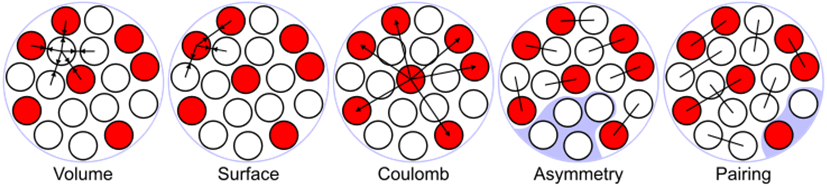Fig. 1. Illustration of the terms of the semi-empirical mass formula in the liquid drop model of the atomic nucleus.

Volume Energy. When an assembly of nucleons of the same size is packed together into the smallest volume, each interior nucleon has a certain number of other nucleons in contact with it. So, this nuclear energy is proportional to the volume.

Surface Energy. A nucleon at the surface of a nucleus interacts with fewer other nucleons than one in the interior of the nucleus and hence its binding energy is less. This surface energy term takes that into account and is therefore negative and is proportional to the surface area.

Coulomb Energy. The electric repulsion between each pair of protons in a nucleus contributes toward decreasing its binding energy.

Asymmetry Energy (also called Pauli Energy). Energy associated with the Pauli Exclusion Principle. Were it not for the Coulomb energy, the most stable form of nuclear matter would have the same number of neutrons as protons, since unequal numbers of neutrons and protons imply filling higher energy levels for one type of particle, while leaving lower energy levels vacant for the other type.

Pairing Energy. An Energy which is a correction term that arises from the tendency of proton pairs and neutron pairs to occur. An even number of particles is more stable than an odd number.

3. The Least Squares Method (LSM)

3.1. History

The method of least squares grew out of the fields of astronomy and geodesy as scientists and mathematicians sought to provide solutions to the challenges of navigating the Earth's oceans during the Age of Exploration. The accurate description of the behavior of celestial bodies was the key to enabling ships to sail in open seas, where sailors could no longer rely on land sightings for navigation.

The method was the culmination of several advances that took place during the course of the eighteenth century :

The combination of different observations as being the best estimate of the true value; errors decrease with aggregation rather than increase, perhaps first expressed by Roger Cotes in 1722.

The combination of different observations taken under the same conditions contrary to simply trying one's best to observe and record a single observation accurately. The approach was known as the method of averages. This approach was notably used by Tobias Mayer while studying the librations of the moon in 1750, and by Pierre-Simon Laplace in his work in explaining the differences in motion of Jupiter and Saturn in 1788.

The combination of different observations taken under different conditions. The method came to be known as the method of least absolute deviation. It was notably performed by Roger Joseph Boscovich in his work on the shape of the earth in 1757 and by Pierre-Simon Laplace for the same problem in 1799.

The development of a criterion that can be evaluated to determine when the solution with the minimum error has been achieved. Laplace tried to specify a mathematical form of the probability density for the errors and define a method of estimation that minimizes the error of estimation. For this purpose, Laplace used a symmetric two-sided exponential distribution we now call Laplace distribution to model the error distribution, and used the sum of absolute deviation as error of estimation. He felt these to be the simplest assumptions he could make, and he had hoped to obtain the arithmetic mean as the best estimate. Instead, his estimator was the posterior median.

The LSM is one of the methods commonly used for data fitness   .

The method of least squares is a standard approach in regression analysis to the approximate solution of over determined systems, i.e., sets of equations in which there are more equations than unknowns.

This allows us to use least squares method for determining the constants in theoretical formulas which are empirical basis.

"Least squares" means that the overall solution minimizes the sum of the squares of the errors made in the results of every single equation.

The most important application is in data fitting. Least squares problems fall into two categories: linear or ordinary least squares and non-linear least squares, depending on whether or not the residuals are linear in all unknowns. The linear least-squares problem occurs in statistical regression analysis; it has a closed-form solution. The non-linear problem is usually solved by iterative refinement; at each iteration the system is approximated by a linear one, and thus the core calculation is similar in both cases.

Polynomial least squares describe the variance in a prediction of the dependent variable as a function of the independent variable and the deviations from the fitted curve. When the observations come from an exponential family and mild conditions are satisfied, least-squares estimates and maximum-likelihood estimates are identical .

The first clear and concise exposition of the method of least squares was published by Legendre in 1805 . The technique is described as an algebraic procedure for fitting linear equations to data and Legendre demonstrates the new method by analyzing the same data as Laplace for the shape of the earth. The value of Legendre's method of least squares was immediately recognized by leading astronomers and geodesists of the time.

In 1809 Carl Friedrich Gauss published his method of calculating the orbits of celestial bodies. In that work he claimed to have been in possession of the method of least squares since 1795. This naturally led to a priority dispute with Legendre. However, to Gauss's credit, he went beyond Legendre and succeeded in connecting the method of least squares with the principles of probability and to the normal distribution  . He had managed to complete Laplace's program of specifying a mathematical form of the probability density for the observations, depending on a finite number of unknown parameters, and define a method of estimation that minimizes the error of estimation. Gauss showed that arithmetic mean is indeed the best estimate of the location parameter by changing both the probability density and the method of estimation. He then turned the problem around by asking what form the density should have and what method of estimation should be used to get the arithmetic mean as estimate of the location parameter. In this attempt, he invented the normal distribution  .

3.2. The Method

General term for each error in the LSM of linear type is a function as: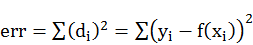(1)

If it is assumed that fit a polynomial function, then: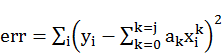(2)

For example, with two columns of data (x, y), the objective is to minimize the error in equation (2), thus: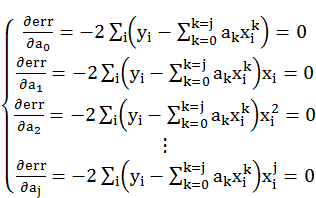(3)

rewriting of equation (3) results in the following: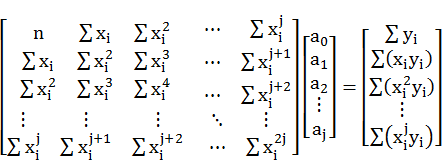(4)

Where i=1, 2,…, n.ak coefficients are indeterminate of the problem in equation (4). Assuming the data in Table 1 to be related to a polynomial with degree of 5, the objective would be to find the coefficients with the best data fitness for a polynomial with degree of 5.

Table 1. Pair of data related to polynomial with degree of 5.

 xi yi -1.4833 39.0336 -1.0667 12.2011 -0.6500 3.5372 -0.2333 1.3652 0.1833 0.8807 0.6000 1.1373 1.0167 2.03245 1.4333 1.2934 1.8500 -8.5361 2.2667 -43.1118

The related matrix equation was changed into the equation (5) after calculation of entries proportionate to equation (4).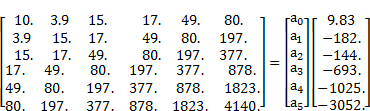(5)

The results were a5=-2.0001, a4=3.0002, a3=-0.9998, a2=1.9994, a1=-1.0001, a0=1.0002. Therefore, the best data fitness for polynomial with degree of 5 for data in Table 1 was equation (6):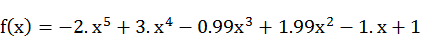(6)

The atomic mass of elements in terms of the energy stored in the nucleus (BE) written in SEMF as below: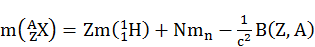(7)

and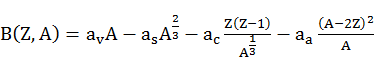(8)

where in (8), A, Z, m(1H) and mn are mass number, atomic number, mass of hydrogen atom, and neutron mass respectively. B(Z,A) is binding energy for a nucleus with mass number A and atomic number Z [equation (8) is just applied and valid for BE of those nuclei with odd mass number in which their numbers of protons or neutrons is none of 2, 8, 20, 28, 50, 82, 126, and 184. These numbers are called "magical numbers" in nuclear Physics, including volume, surface, coulomb, and asymmetry terms respectively. Coefficient of volume term (ay), surface term (as), coulomb term (ac), and asymmetry term (aa) were calculated by LSM (Table 2).

Generalizing LSM to this problem results in definition of function (9):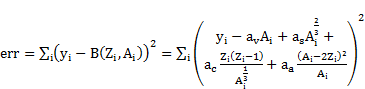(9)

If the above-mentioned function is minimized, then the coefficient of volume, surface, coulomb, and asymmetry terms are obtained; in other words, partial derivative of function err in proportion to coefficient of volume term (ay), surface term(as), coulomb term(ac), and asymmetry term (aa) should equal zero.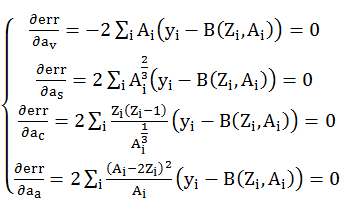(10)

In equation (10), yi is binding energy of i-th special nucleus obtained from equation (8) when mass of hydrogen atom and neutron be given.

Data of Table 2 was selected in a way that the share of parity term equals zero.

Table 2. Symbol, atomic number, mass number, and atomic mass of some special nucleus .

 atomic mass (m) in terms of u mass number atomic number Nucleus symbol 13.003355 13 6 C 20.993843 21 10 Ne 20.997651 21 11 Na 26.986704 27 14 Si 34.969032 35 16 S 34.975256 35 18 Ar 40.961825 41 19 K 44.958124 45 22 Ti 52.944340 53 23 V 50.948213 51 25 Mn 60.933461 61 29 Cu 80.923270 81 38 Sr 102.906323 103 44 Ru 116. 908630 117 52 Te 142.910930 143 61 Pm 182.950817 183 75 Re 182.953290 183 76 Os 192.966560 193 80 Hg 206.980456 207 84 Po 215.000310 215 87 Fr 235.043924 235 92 U 251.079580 251 98 Cf

System of equation (10) results in matrix equation 11: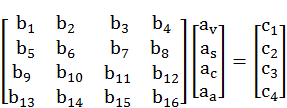(11)

where,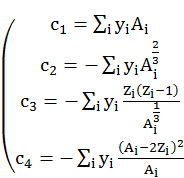(12)

and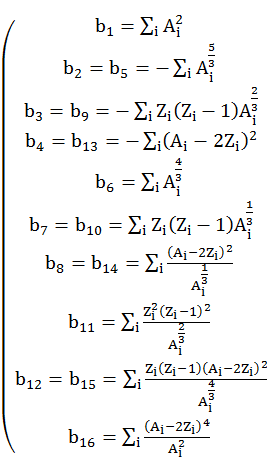(13)

The required determinants were c2=931.494061 MeV/u, mass of hydrogen atom=1.007940u, and neutron mass=1.008665u. The results obtained through the LSM for volume, surface, coulomb, and asymmetry coefficients were av=15.519MeV، as=17.476MeV، ac=0.674MeV and aa=24.576MeV respectively.

4. Conclusion and Recommendation

Comparing the results obtained for volume, surface, coulomb, and asymmetry terms with those of other studies showed an appropriate compatibility. It is recommended to use more input data with higher accuracy obtained from more recent references, so as to increase the accuracy of the results. An estimation of neutron and hydrogen atom masses can be presented if their masses in equation (7) are regarded indeterminate.

References

1. Bailey, D. "Semi-empirical Nuclear Mass Formula". PHY357: Strings & Binding Energy. University of Toronto. Retrieved 2011-03-31.
2. Weizsäcker, CF V. "Zur theorie der kernmassen." Zeitschrift für Physik A Hadrons and Nuclei 96.7 (1935): 431-458.
3. Smagulov, Samat, and Vadim Logachev. "Nuclear Tests in the USSR: The Red Book (From Nuclear History: Fear, Horror and Nuclear Blackmail) Anatoliy Matushchenko Co-Chairman of the Interagency Expert Commission under the Scientific Research Institute for Pulse Engineering, and Advisor to." Energy, Society and Security (2008): 405.
4. Wyler, John Archibald. "Rasputin, science, and the transmogrification of destiny." General Relativity and Gravitation 5.2 (1974): 175-182.
5. Bethe, Hans Albrecht, and Robert Fox Bacher. "Nuclear physics A. Stationary states of nuclei." Reviews of Modern Physics 8.2 (1936): 82.
6. Bleck-Neuhaus, Jörn. Elementare Teilchen: moderne Physik von den Atomen bis zum Standard-Modell. Springer-Verlag, 2010.
7. Derrick, Malcolm, et al. "Measurement of total and partial photon proton cross sections at 180 GeV center of mass energy." Zeitschrift für Physik C Particles and Fields 63.3 (1994): 391-408.
8. Myers, William D. Droplet model of atomic nuclei. Ifi/Plenum, 1977.
9. Sobiczewski, Adam. "Progress in theoretical understanding of properties of the heaviest nuclei." Physics of particles and nuclei 25.2 (1994): 119-128.
10. Davidson, N. J., et al. "A semi-empirical determination of the properties of nuclear matter." Nuclear Physics A 570.1 (1994): 61-68.
11. Charnes, A.; Frome, E. L.; Yu, P. L. (1976). "The Equivalence of Generalized Least Squares and Maximum Likelihood Estimates in the Exponential Family". Journal of the American Statistical Association 71 (353): 169–171.
12. Stigler, Stephen M. The history of statistics: The measurement of uncertainty before 1900. Harvard University Press, 1986.
13. Legendre, Adrien Marie. Nouvelles méthodes pour la détermination des orbites des comètes. No. 1. F. Didot, 1805.
14. Martin, James E. Physics for radiation protection. John Wiley & Sons, 2013.
15. Stigler, Stephen M. (1986). The History of Statistics: The Measurement of Uncertainty Before 1900. Cambridge, MA: Belknap Press of Harvard University Press. ISBN 0-674-40340-1.
16. Legendre, Adrien-Marie (1805), Nouvelles méthodes pour la détermination des orbites des comètes [New Methods for the Determination of the Orbits of Comets] (in French), Paris: F. Didot.
17. Zeidler, Eberhard. Oxford users' guide to mathematics. Oxford University Press, 2004.
18. Dunnington, Waldo. "The Sesquicentennial of the Birth of Gauss." The Scientific Monthly 24 (1927): 402-414.
19. McGurrin, Danielle, et al. "White collar crime representation in the criminological literature revisited, 2001-2010." W. Criminology Rev. 14 (2013): 3.
20. Wallace, Hon Alfred R., and Esteemed Master. "Unique WCP identifier: WCP2516. 2406.".

 Contents 1. 2. 3. 3.1. 3.2. 4.
Article ToolsAbstractPDF(458K)